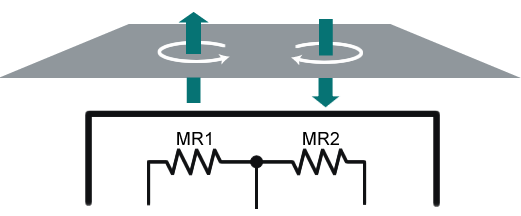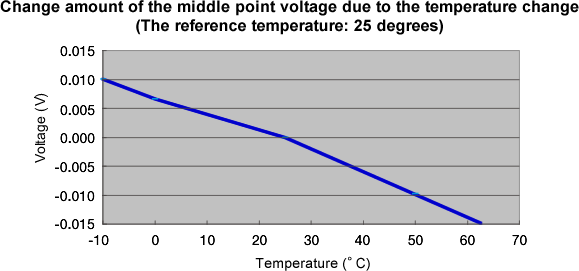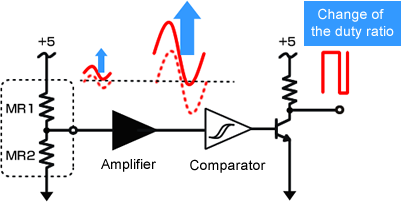MP series FAQWhy the duty ratio of the MP-981 Magneto-electric rotation detector changes?Change of the duty ratio (Rotation speed dependence)

When the magnetic body is moved through the magnetic field, the eddy current is generated in the surface, and magnetic field occurs (green arrow in the following figure). When this magnetic field interferes with the magnetic field which is obtained by the magnetic resistance sensor, the middle point voltage is changed.The direction of the eddy current is changed by the movement direction of the magnetic body. Moreover, since the eddy current is increased in proportion to the speed, the change of the middle point voltage is increased, when the rotation speed is increased.

Change of the duty ratio (Temperature dependence)

The magnetic resistance element has the temperature dependency. Because the MP-981 is using two magnetic resistance elements, the middle point voltage is changed depending on the slight difference of the temperature characteristics between them. The following figure is an example of it.Change of the middle point voltage output

Waveform-shaped signal which is obtained by the magnetic resistance sensor is set as the final signal. The signal amplitude which can be obtained by the magnetic resistance sensor is very small such as tens mV, so when the middle point voltage is changed by the temperature change or increase of the rotation speed, the change of the duty ratio or lost of the pulse output may occur.Revised:2013.06.17